# Using ‘shapviz’

## Overview

SHAP (SHapley Additive exPlanations, see Lundberg and Lee (2017)) is an ingenious way to study black box models. SHAP values decompose - as fair as possible - predictions into additive feature contributions. Crunching SHAP values requires clever algorithms by clever people. Analyzing them, however, is super easy with the right visualizations. {shapviz} offers the latter:

• sv_importance(): Importance plots (bar plots and/or beeswarm plots) to study variable importance.
• sv_dependence() and sv_dependence2D(): Dependence plots to study feature effects and interactions.
• sv_interaction(): Interaction plots.
• sv_waterfall(): Waterfall plots.
• sv_force(): Force plots as an alternative to waterfall plots.

These plots require a “shapviz” object, which is built from two things only:

1. S: Matrix of SHAP values
2. X: Dataset that includes the corresponding feature values

Optionally, a baseline can be passed to represent an average prediction on the scale of the SHAP values. Also a 3D array of SHAP interaction values can be passed as S_inter.

A key feature of “shapviz” is that X is used for visualization only. Thus it is perfectly fine to use factor variables, even if the underlying model would not accept these. Additionally, in order to improve visualization, it can sometimes make sense to clip gross outliers, take logarithms for certain columns, or replace missing values by some explicit value.

To further simplify the use of {shapviz}, we added direct connectors to:

For XGBoost, LightGBM, and H2O, the SHAP values are directly calculated from the fitted model.

CatBoost is not included, but see Section “Any other package” how to use its SHAP calculation backend with {shapviz}.

See vignette “Multiple shapviz objects” for how to deal with multiple models or multiclass models.

## Installation

# From CRAN
install.packages("shapviz")

# Or the newest version from GitHub:
# install.packages("devtools")
devtools::install_github("ModelOriented/shapviz")

## Usage

### Fit model on diamond prices

We start by fitting an XGBoost model to predict diamond prices based on the four “C” features.

library(shapviz)
library(ggplot2)
library(xgboost)

set.seed(3653)

# Turn ordinal factors into normal ones (required for some of the examples)
ord <- c("clarity", "cut", "color")
diamonds[, ord] <- lapply(diamonds[, ord], factor, ordered = FALSE)

# Fit XGBoost model
x <- c("carat", "clarity", "cut", "color")
dtrain <- xgb.DMatrix(data.matrix(diamonds[x]), label = diamonds$price) fit <- xgb.train(params = list(learning_rate = 0.1), data = dtrain, nrounds = 65) ### Create “shapviz” object One line of code creates a “shapviz” object. It contains SHAP values and feature values for the set of observations we are interested in. Note again that X is solely used as explanation dataset, not for calculating SHAP values. In this example we construct the “shapviz” object directly from the fitted XGBoost model. Thus we also need to pass a corresponding prediction dataset X_pred used for calculating SHAP values by XGBoost. # Explanation data dia_small <- diamonds[sample(nrow(diamonds), 2000), ] shp <- shapviz(fit, X_pred = data.matrix(dia_small[x]), X = dia_small) Note: If X_pred would contain one-hot-encoded dummy variables, their SHAP values (and also SHAP interaction values) could be collapsed by the collapse argument of shapviz(). ### Decompose single prediction The main idea behind SHAP values is to decompose, in a fair way, a prediction into additive contributions of each feature. Typical visualizations include waterfall plots and force plots: sv_waterfall(shp, row_id = 1) + theme(axis.text = element_text(size = 11))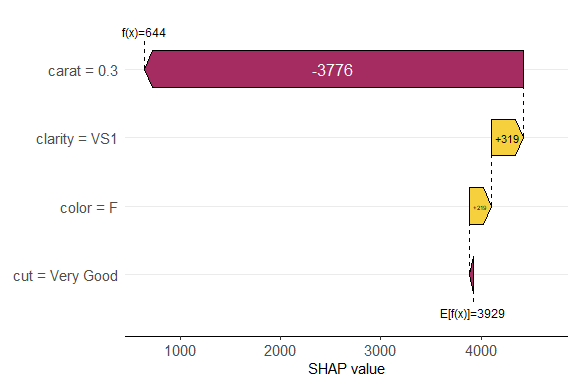Works pretty sweet, and factor input is respected! Alternatively, we can study a force plot: sv_force(shp, row_id = 1)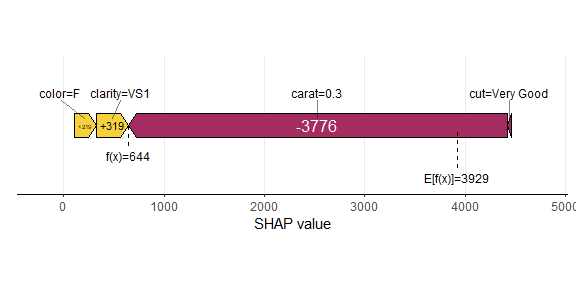Also multiple row_id can be passed: The SHAP values of the selected observations are averaged and then plotted as aggregated SHAP values: The prediction profile for beautiful color “D” diamonds: sv_waterfall(shp, shp$X$color == "D") + theme(axis.text = element_text(size = 11))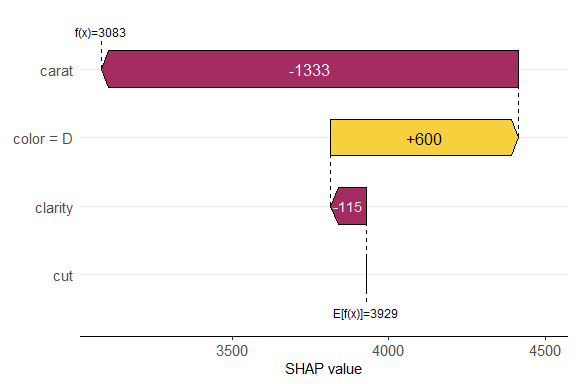### SHAP importance We have decomposed 2000 predictions, not just one. This allows us to study variable importance at a global model level by studying average absolute SHAP values or by looking at beeswarm “summary” plots of SHAP values. # A barplot of mean absolute SHAP values sv_importance(shp)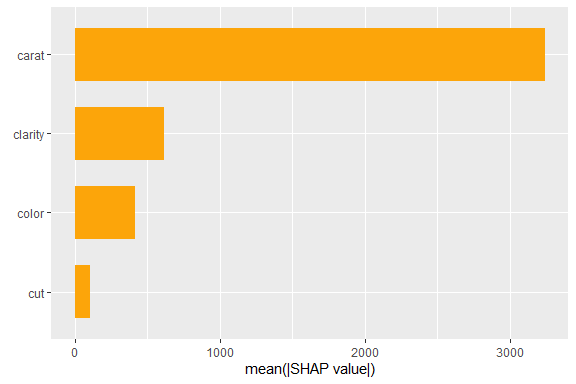# A beeswarm plot sv_importance(shp, kind = "beeswarm")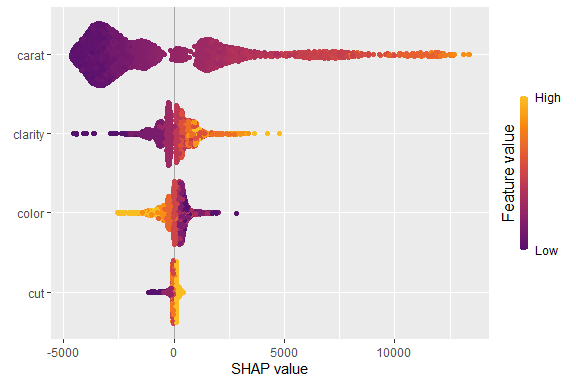# Or both! sv_importance(shp, kind = "both", show_numbers = TRUE, bee_width = 0.2)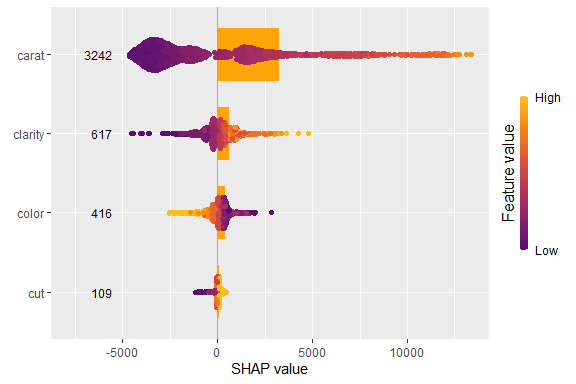### SHAP dependence plots A SHAP beeswarm importance plot gives first hints on whether high feature values tend to high or low predictions. This impression can be substantiated by studying simple scatterplots of SHAP values of a feature against its feature values. On the color axis, the feature with (heuristically) strongest interaction is shown by default. Use color_var to use another feature (or NULL for no coloring). sv_dependence(shp, v = "color")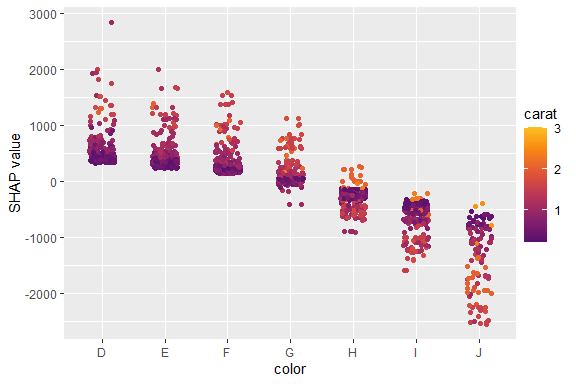sv_dependence(shp, v = "carat", alpha = 0.2, size = 1) + guides(colour = guide_legend(override.aes = list(alpha = 1, size = 2)))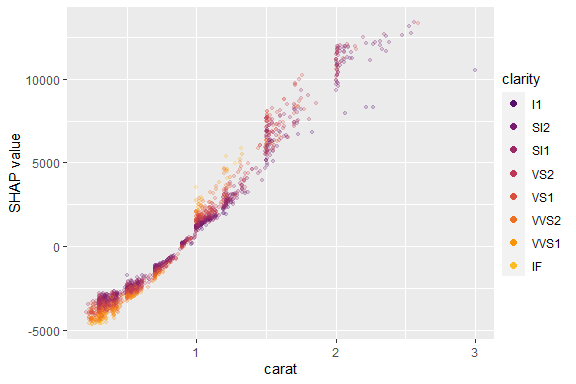Using {patchwork}, we can also plot multiple features at the same time. library(patchwork) # to use the & operator sv_dependence(shp, v = x) & theme_gray(base_size = 9) & ylim(-5000, 15000)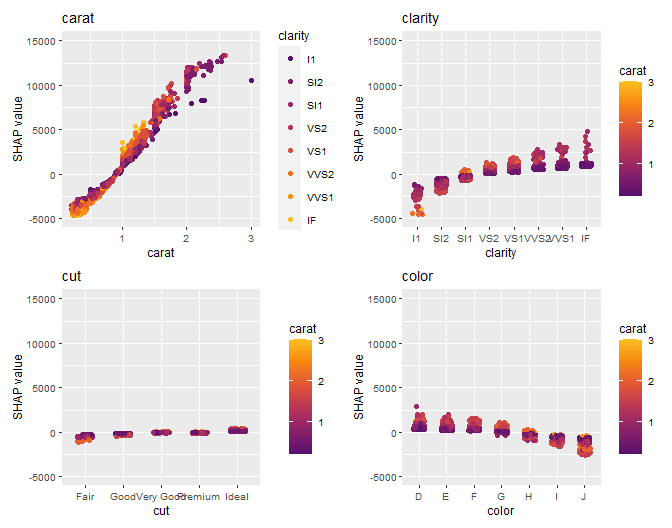To show the combined effects of two features (sum of their SHAP values), 2-dimensional dependence plots are available: sv_dependence2D(shp, x = "carat", y = c("clarity", "color"), alpha = 0.5)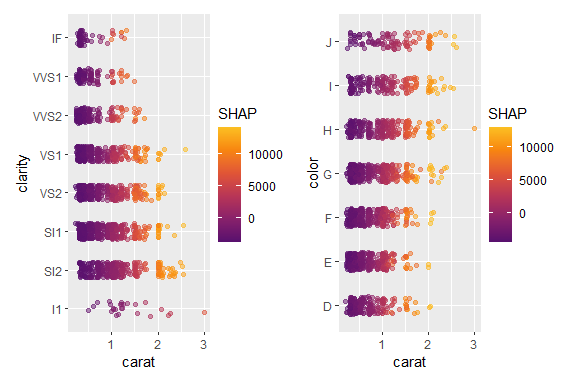This is especially interesting for geographic components in a model. ### Interactions If SHAP interaction values have been computed (via {xgboost} or {treeshap}), the dependence plot can focus on main effects or SHAP interaction effects (multiplied by two due to symmetry): shp_i <- shapviz( fit, X_pred = data.matrix(dia_small[x]), X = dia_small, interactions = TRUE ) sv_dependence(shp_i, v = "color", color_var = "cut", interactions = TRUE)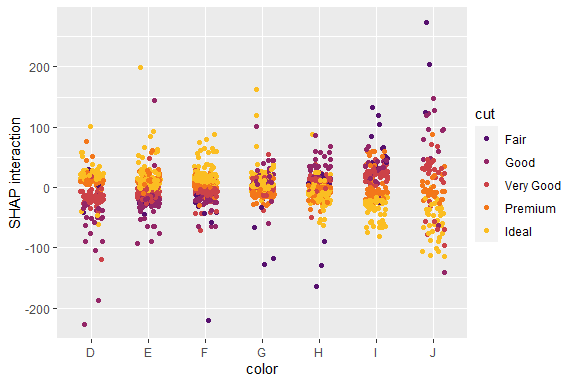Using {patchwork}, we can show multiple colors at the same time: sv_dependence(shp_i, v = "carat", color_var = x, interactions = TRUE) & ylim(-6000, 13000)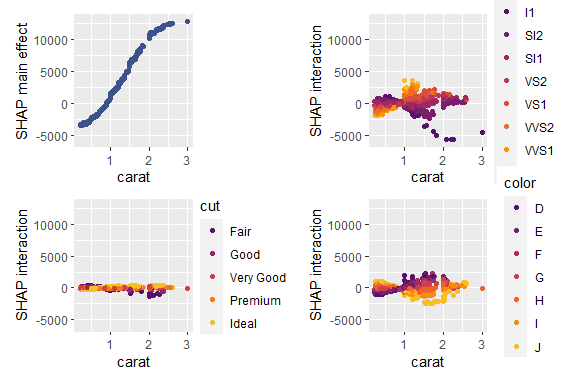We can also study all interactions and main effects together using the following beeswarm visualization: sv_interaction(shp_i) + theme(axis.text.x = element_text(angle = 45, vjust = 1, hjust = 1))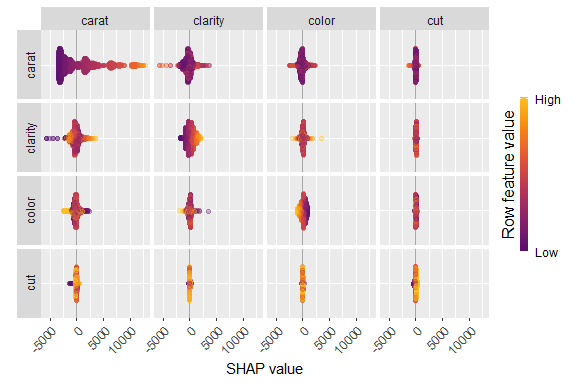## Interface to other packages The above example uses XGBoost to calculate SHAP values. In the following sections, we show (without running the code), how other packages work together with {shapviz}. ### LightGBM library(lightgbm) dtrain <- lgb.Dataset(data.matrix(diamonds[x]), label = diamonds$price)

fit <- lgb.train(
params = list(learning_rate = 0.1, objective = "regression"),
data = dtrain,
nrounds = 65
)

shp <- shapviz(fit, X_pred = data.matrix(dia_small[x]), X = dia_small)
sv_importance(shp)

### fastshap

library(fastshap)

fit <- lm(Sepal.Length ~ Sepal.Width + Petal.Length, data = iris)

# fastshap > 0.0.7 and shap_only = FALSE
shap <- fastshap::explain(
fit,
X = iris[c("Sepal.Width", "Petal.Length")],
nsim = 100,
pred_wrapper = predict,
shap_only = FALSE
)

sv <- shapviz(shap)
sv_dependence(sv, "Sepal.Width", color_var = NULL)

# fastshap <= 0.0.7 or shap_only = TRUE
shap <- fastshap::explain(
fit,
X = iris[c("Sepal.Width", "Petal.Length")],
nsim = 100,
pred_wrapper = predict
)

sv <- shapviz(shap, X = iris, baseline = mean(iris$Sepal.Length)) sv_dependence(sv, "Sepal.Width", color_var = NULL) ### shapr library(shapr) fit <- lm(price ~ carat + clarity + cut + color, data = diamonds) background <- diamonds[sample(nrow(diamonds), 100), ] explainer <- shapr(background, fit) explanation <- shapr::explain( as.data.frame(dia_small)[1:200, ], approach = "ctree", explainer = explainer, prediction_zero = mean(diamonds$price)
)

shp <- shapviz(explanation)
sv_dependence(shp, "carat")

### H2O

If you work with a tree-based H2O model:

library(h2o)

h2o.init()

dia_h2o <- as.h2o(diamonds)

fit <- h2o.gbm(x, "price", training_frame = dia_h2o)
shp <- shapviz(fit, X_pred = dia_small)
sv_force(shp, row_id = 1)
sv_dependence(shp, "carat")

### treeshap

library(treeshap)
library(ranger)

fit <- ranger(
y = diamonds$price, x = data.matrix(diamonds[x]), max.depth = 6, num.trees = 100 ) unified_model <- ranger.unify(fit, data.matrix(diamonds[x])) shaps <- treeshap(unified_model, data.matrix(dia_small[x]), interactions = TRUE) shp <- shapviz(shaps, X = dia_small) sv_importance(shp) sv_dependence(shp, "carat", alpha = 0.7, interactions = TRUE) ### DALEX Decompositions of single predictions obtained by DALEX::predict_parts(): library(DALEX) library(ranger) fit <- ranger(reformulate(x, "price"), data = diamonds, max.depth = 6, num.trees = 100) explainer <- DALEX::explain(fit, data = diamonds[x], y = diamonds$price, label = "RF")
breakdown <- predict_parts(explainer, diamonds[10000, ], keep_distributions = FALSE)

sv_force(shapviz(breakdown)) +
xlab("Breakdown")

### kernelshap

library(kernelshap)

background <- diamonds[sample(nrow(diamonds), 200), ]
fit <- lm(price ~ carat + clarity + cut + color, data = diamonds)
ks <- kernelshap(fit, dia_small[x], bg_X = background)

shp <- shapviz(ks)
sv_importance(shp)
sv_dependence(shp, "carat", color_var = NULL)

### Any other package

The most general interface is to provide a matrix of SHAP values and corresponding feature values (and optionally, a baseline value):

S <- matrix(c(1, -1, -1, 1), ncol = 2, dimnames = list(NULL, c("x", "y")))
X <- data.frame(x = c("a", "b"), y = c(100, 10))
shp <- shapviz(S, X, baseline = 4)

An example is CatBoost: it is not on CRAN and requires catboost.*() functions to calculate SHAP values, so we cannot directly add it to {shapviz} for now. Just use a wrapper like this:

library(catboost)

shapviz.catboost.Model <- function(object, X_pred, X = X_pred, collapse = NULL, ...) {
if (!requireNamespace("catboost", quietly = TRUE)) {
stop("Package 'catboost' not installed")
}
stopifnot(
"X must be a matrix or data.frame. It can't be an object of class catboost.Pool" =
is.matrix(X) || is.data.frame(X),
"X_pred must be a matrix, a data.frame, or a catboost.Pool" =
is.matrix(X_pred) || is.data.frame(X_pred) || inherits(X_pred, "catboost.Pool"),
"X_pred must have column names" = !is.null(colnames(X_pred))
)

if (!inherits(X_pred, "catboost.Pool")) {
}

S <- catboost.get_feature_importance(object, X_pred, type = "ShapValues", ...)

# Call matrix method
pp <- ncol(X_pred) + 1
baseline <- S[1, pp]
S <- S[, -pp, drop = FALSE]
colnames(S) <- colnames(X_pred)
shapviz(S, X = X, baseline = baseline, collapse = collapse)
}

# Example
X_pool <- catboost.load_pool(diamonds[x], label = diamonds\$price)

fit <- catboost.train(
X_pool,
params = list(
loss_function = "RMSE",
iterations = 165,
logging_level = "Silent",
allow_writing_files = FALSE
)
)

shp <- shapviz(fit, X_pred = dia_small[x])
sv_importance(shp)
sv_dependence(shp, "clarity", alpha = 0.2, size = 1)